# We Helped With This R Language Programming Assignment: Have A Similar One?

SOLVEDCategory Programming R | R Studio Undergraduate Solved Statistics Assignment Experts

## Assignment Description

Homework #3 Scatterplots and Correlation

A sample of 24 faculty members at WMU was conducted and each professor was asked their age and annual income. The results of this study are listed below:

 Person Age (X) Income (Y) (x \$1000) 1 40 32 2 31 24 3 50 47 4 53 50 5 36 30 6 55 55 7 37 33 8 45 41 9 60 63 10 41 34 11 46 43 12 38 35 13 32 28 14 56 57 15 51 50 16 37 30 17 54 52 18 42 35 19 47 41 20 33 26 21 39 34 22 52 49 23 57 60 24 55 51

a.        This data is in the FacSalaries.csv file on the eLearning page for GEOG 5670 in the data section — copy this to your USB drive in a folder called “Correlations.” In RStudio navigate to this folder and make it the working directory. Load this into a dataframe called Salaries using the read.csv() function.

b.       The first step in any analysis of correlations is to generate a scatterplot. Install and load the ggplot2 package and use qplot() to generate a simple scatterplot. Specify the Age data for the x axis and the Salary data for the y. Set the geom= parameter equal to “point”. Specify appropriate labels for the x axis and y axis using the xlab= and ylab= arguments, and use main= to give the plot an appropriate title. In RStudio click on the Plot tab and Export button to save this to the clipboard. Paste this into a Word document.

c.        Compute the covariance between Age and Income using the cov() function. Copy the output from this and paste it below the scatterplot in the Word document. Answer the following questions in the Word document:

1.       What does the sign (+ or -) indicate?

2.       If the salaries were expressed as dollars instead of the current \$ x 1000, how do you think the value of the covariance would change?

d.       Now use the cor.test() function to determine the correlation between the two variables. You can specify a fomula as: ~ Age + Income, data = Salaries and be sure to set the method to “pearson” Copy the output from this and paste it to the Word document beneath the covariance. Rerun this command with the methods set to “spearman” and “kendall”. Copy the output from these to your Word document. Answer the following questions:

1.       Does correlation have more or less utility in determining the strength of the linear relationship between Age and Income? Briefly explain why.

2.       If we had an additional ordinal variable that indicates the rank of each professor (Instructor, Assistant Professor, Associate Prof, Professor), could we use Pearson’s r to measure the strength of the relationship between Age and Income?

3.       How do the values of the three methods (Pearson, Spearman, and Kendall) compare?

1.       This question uses agricultural data from China provided by Dr. Veeck. Copy the ChinaAgCorr.csv file from the Data section of the GEOG 5670 elearning page and paste it in your Correlation folder. Import this to a data frame called ChinaAg.

The variables in this dataset are: MECHIDX-an index of the amount of mechanization in ag.; AGCHEM—the amount of ag. chemicals used in each district; ECOCROPS—the area of ecologically benign crops (such as nuts) under cultivation; DIVERSIT- an index of the biodiversity of the district; TOTFRMS—the total number of farms; AGAREA—the total area under cultivation; ARABLE—the total area of arable land; IRRIG—the total area of irrigated land.

2.       A quick way to generate a matrix of scatterplots for all combinations of these eight variable is the pairs() function. Run this on the ChinaAg dataframe and copy the resulting graph to your Word document. Using the combinations below the diagonal names on this graph, indicate which combinations are positively related, negatively related, or weakly related and list these below the graph in your Word document.

3.       This time, we’ll use the cor() function on the whole dataframe to get a correlation matrix. You can make a cleaner output for this matrix if you round the values of the correlation coefficient to 3 decimal places using the round() function. So nest the cov() function inside the round() function to accomplish this. Copy the output matrix and paste this in your Word document.

Print out your Word document and turn it in for credit on the homework ass

GEOG 5670 Spatial Analysis                                                 Name:  ______________________

Homework #3

Scatterplots and Correlation

Paste scatterplot of Age vs. Income data:

Paste covariance output here:

1.      What does the sign (+ or -) indicate?

2.      If  the salaries were expressed as dollars instead of the current \$ x 1000, how do you think the value of the covariance would change?

Paste Pearson’s r output here:

3.      What is the value for Pearson’s r?  Briefly state what this means.

4.      Does this value indicate a significantly significant correlation?  What evidence can you cite from the output?

5.      As compared to the covariance value computed earlier, does correlation have more or less utility in determining the strength of the linear relationship between Age and Income?  Briefly explain why.

6. Paste ChinaAg scatterplot matrix here:

Pairs that are + correlated                Pairs that are - correlated       Pairs with weak correlation

7. Paste the correlation matrix here:

## Assignment Description

CORRELATION

### Preview

Bivariate Random Variables

Correlation Analysis

Pearson’s r

Spatial Autocorrelation

Regression Analysis

Linear Regression

Goodness of Fit

Homework #5 is on the course web page—try to finish by March 12

####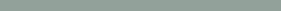Multivariate Techniques

Involve two or more variables

Covariance of Two Random Variables

𝑁

Simple (bivariate) correlation analysis is an investigation

of the strength of association between two variables

Simple regression analysis is a study of the nature of the relationship

Estimating the value of one variable given another

1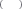𝐶𝑜𝑣 𝑋, 𝑌 = 𝑁 − 1

•  C(X,Y) is the covariance

𝑋𝑖 𝑋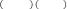𝑖=1

𝑌𝑖  𝑌

Values of 0 indicate no relationship

If X increases result in increases in Y,  + values

•  If X increases result in decreases in Y,  - values

Problem with covariance is that it is in units of X and Y, so values are difficult to interpret#### Sample Covariance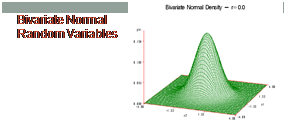The best point estimate for C(X,Y) isS     =      ån    ( X  - X )(Y  - Y )

XY       n -1         i                i i=1

##### • SXY is positive if the two variables have a positive relationship and it is negative if they are negatively related

Sample covariance has the same disadvantage as

C(X,Y)

It is highly influenced by the units in which the two variables are measured

##### • If two random variables are jointly normally distributed

The marginal distributions of both X and Y are univariate normal

Any conditional distribution of X or Y is also univariate normal

mx my sx sy rxy

rxy

= C( X ,Y )

o s

x y

#### Pearson’s Product Moment Correlation Coefficient

Population parameters mx my sx sy rxy are almost never

#### Sample Correlation Coefficient

Substitutes appropriate point estimators for C(X,Y) into

known

Must estimate rxy from sample data

In simplified form

rxy

= C( X ,Y )

#### • Pearson’s r is the best point estimate of rxy

Where all points plot on a positively sloped line, r = 1

Where all points plot on a negatively sloped line, r = -1

If r is near 0, the scatter of points is nearly circular

A scatter of points can have a strong nonlinear association but still have r near 0

x y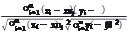𝑟 =

####Scatter Diagrams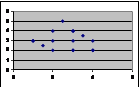Each observation pair (Xi,Yi) represents one dot

### Positive Linear Association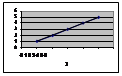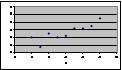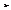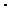r = 1 if all points lie on a positively sloped line

r = 0.88 in the second example###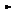Negative Linear Association

 Real Estate Hair Height, in Income (\$ x 1000) 4.5 100 6 130 5.5 160 7 180 7.5 190 8 200 10 220 9 240 10.5 280 12 300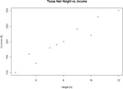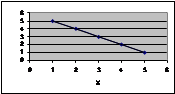r = -1 if all points lie on a negatively sloped line

We want to see if there is a relationship between height of hair and income from real estate

### Calculation of Pearson’s r

 Xi Yi Xi^2 Yi^2 XiYi 4.5 100 20.25 10000 450 6.0 130 36.00 16900 780 5.5 160 30.25 25600 880 7.0 180 49.00 32400 1260 7.5 190 56.25 36100 1425 8.0 200 64.00 40000 1600 10.0 220 100.00 48400 2200 9.0 240 81.00 57600 2160 10.5 280 110.25 78400 2940 12.0 300 144.00 90000 3600 80 2000 691 435400 17295

Covariance and Correlation in R

Both in base package

cov() or cor()

cov(x, y = NULL, use = c("everything", “all.obs”, “complete.obs”), method = c("pearson", "kendall", "spearman"))

cor(x, y = NULL, use = c("everything", “all.obs”, “complete.obs”), method = c("pearson", "kendall", "spearman"))

cor.test()

cor.test(x, y, alternative = c("two.sided", "less", "greater"), method =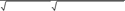r =             17295 - (80)(2000) /10

691- 802 /10 435400 - 20002 /10

= 0.96

c("pearson", "kendall", "spearman"), exact = NULL, conf.level = 0.95, continuity = FALSE, ...)

cor() can do all correlations in a dataframe while cor.test()

There is a strong positive correlation between hair height

and real estate sales--higher hair = more real estate sales

only does specified pairs of variables

####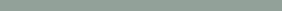Significance Testing

Assume two random variables are bivariate

normally distributed

H0: r = 0, HA: r ¹ 0, or r > 0 or r < 0

1- r 2

sr   =    n - 2

#### •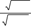The test statistic is:

Correlation Matrices

A summary of the correlation coefficients between all pairs of variables in a set

If for the Texas example we had data on

Hair size

Real Estate Income

Percentage of gold chrome on car

Tons of makeup applied annually

Numbers of packs of More cigarettes smoked per day

Monthly bill for Home Shopping Networkt = r =

Sr

r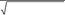(1- r 2 ) /(n - 2)

= r n - 2 1- r 2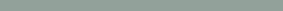###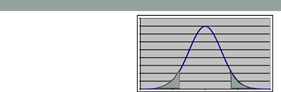Correlation MatrixforTexas                                                  TexasExample

Hair

Real Estate Gold Chrome Makeup

More Cigs HSN Bill

Hair Real Estate Gold Chrome Makeup More Cigs HSN

 1 0.96 0.83 0.91 -0.53 0.92 0.96 1 0.98 0.8 0.23 0.91 0.83 0.98 1 0.62 -0.71 0.94 0.91 0.8 0.62 1 0.07 0.21 -0.53 0.23 -0.71 0.07 1 -0.77 0.92 0.91 0.94 0.21 -0.77 1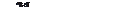t = -2.306         0         2.306 10.2

> cor.test(~HairHgt + Income, data=TexasHair, method="pearson")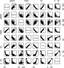You can also generate a matrix of scatterplots using the pairs() command in the R graphics package

Pearson's product-moment correlation data: HairHgt and Income

t = 10.223, df = 8, p-value = 7.198e-06

alternative hypothesis: true correlation is not equal to 0

95 percent confidence interval: 0.8499218 0.9916539

sample estimates:

cor

0.9637914

#### General Procedure for Correlations Using

R

To compute basic correlation coefficients there are three main functions that can be used:

cor(), cor.test() and rcorr().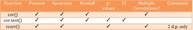### Pearson Correlation Output

Exam           Anxiety Revise Exam    1.0000000 -0.4409934  0.3967207

Anxiety -0.4409934  1.0000000 -0.7092493

Revise   0.3967207 -0.7092493  1.0000000

### Correlations using R

Pearson correlations:

cor(examData, use = "complete.obs", method = "pearson")

rcorr(examData, type = "pearson")

cor.test(examData\$Exam, examData\$Anxiety, method = "pearson")

If we predicted a negative correlation:

cor.test(examData\$Exam, examData\$Anxiety, alternative = "less"), method = "pearson")### Reporting the Results

Exam performance was significantly correlated with exam anxiety, r = -.44, and time spent revising, r = .40; the time spent revising was also correlated with exam anxiety, r =

-.71 (all ps < .001).

####Things to Know about the Correlation

It varies between -1 and +1

0 = no relationship

It is an effect size

±.1 = small effect

±.3 = medium effect

±.5 = large effect

Coefficient of determination, r2

By squaring the value of r you get the proportion of variance in one variable shared by the other.

### Correlation and Causality

The third-variable problem:

In any correlation, causality between two variables cannot be assumed because there may be other measured or unmeasured variables affecting the results.

Direction of causality:

Correlation coefficients say nothing about which variable causes the other to change.

### Non-parametric Correlation

Spearman’s rho

Pearson’s correlation on the ranked data

Kendall’s tau

Better than Spearman’s for small samples

World’s Biggest Liar competition

68 contestants

Measures

Where they were placed in the competition (first, second, third, etc.)

Creativity questionnaire (maximum score 60)

#### Spearman’s Rho

cor(liarData\$Position, liarData\$Creativity, method =

"spearman")

The output of this command will be:

 -0.3732184

To get the significance value use rcorr() (NB: first convert the dataframe to a matrix):

liarMatrix<-as.matrix(liarData[, c("Position", "Creativity")]) rcorr(liarMatrix)

Or:

cor.test(liarData\$Position, liarData\$Creativity, alternative = "less", method = "spearman")

####Spearman's Rho

Output

Spearman's rank correlation rho

data: liarData\$Position and liarData\$Creativity S = 71948.4, p-value = 0.0008602

alternative hypothesis: true rho is less than 0 sample estimates:

rho

-0.3732184

### Kendall’s Tau (Non-parametric)

To carry out Kendall’s correlation on the World’s Biggest Liar data simply follow the same steps as for Pearson and Spearman correlations but use method = “kendall”:

cor(liarData\$Position, liarData\$Creativity, method = "kendall")

cor.test(liarData\$Position, liarData\$Creativity, alternative = "less", method = "kendall")

####Kendall’s Tau (Non-parametric)

The output is much the same as for Spearman’s correlation.

Kendall's rank correlation tau

data: liarData\$Position and liarData\$Creativity z = -3.2252, p-value = 0.0006294

alternative hypothesis: true tau is less than 0

sample estimates:

tau

-0.3002413

### Bootstrapping Correlations

If we stick with our World’s Biggest Liar data and want to bootstrap Kendall’s tau, then our function will be:

bootTau<-function(liarData,i) cor(liarData\$Position[i], liarData\$Creativity[i], use = "complete.obs", method = "kendall")

To bootstrap a Pearson or Spearman correlation you do it in exactly the same way except that you specify method = “pearson” or method = “spearman” when you define the function.

### Bootstrapping Correlations Output

To create the bootstrap object, we execute:

library(boot)

boot_kendall<-boot(liarData, bootTau, 2000) boot_kendall

To get the 95% confidence interval for the

boot_kendall object:

boot.ci(boot_kendall)

### Bootstrapping Correlations

To bootstrap a Pearson or Spearman correlation you do it in exactly the same way except that you specify method = “pearson” or method = “spearman” when you define the function.

####Bootstrapping Correlations Output

The output below shows the contents of

boot_kendall:

ORDINARY NONPARAMETRIC BOOTSTRAP

Call:

boot(data = liarData, statistic = bootTau, R = 2000)

Bootstrap Statistics :

original bias std. error

t1* -0.3002413 0.001058191 0.097663

### Bootstrapping Correlations Output

The output below shows the contents of the boot.ci()

function:

BOOTSTRAP CONFIDENCE INTERVAL CALCULATIONS

Based on 2000 bootstrap replicates

CALL :

boot.ci(boot.out = boot_kendall)

Intervals :

Level      Normal               Basic

95% (-0.4927, -0.1099 ) (-0.4956, -0.1126 )

Level     Percentile             BCa

95% (-0.4879, -0.1049 ) (-0.4777, -0.0941 )

####Partial and Semi-partial Correlations

Partial correlation:

Exam Performance1

Measures the relationship between two variables, controlling for the effect that a third variable has on them both.

Variance Accounted for by Exam Anxiety (19.4%)

Exam Anxiety

Exam

#### • Semi-partial correlation:

Measures the relationship between two variables controlling for the effect that a third variable has on only one of the others.

2

Variance Accounted for by Revision Time (15.7%)

 3

Variance accounted for by both Exam Anxiety and Revision Time

Performance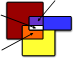Exam Performance

Revision Time

Unique variance accounted for by Revision Time

Revision Time

Unique variance accounted for by Exam Anxiety

Exam Anxiety

####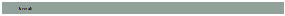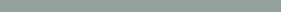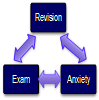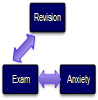Doing Partial Correlation using R

The general form of pcor() is:

pcor(c("var1", "var2", "control1", "control2" etc.), var(dataframe))

We can then see the partial correlation and the value of

R2 in the console by executing:

pc

pc^2

#### PartialCorrelation               Semi-PartialCorrelationDoing Partial Correlation using R

The general form of pcor.test() is:

pcor(pcor object, number of control variables, sample size)

Basically, you enter an object that you have created with pcor() (or you can put the pcor() command directly into the function):

pcor.test(pc, 1, 103)

### Partial Correlation Output

> pc

 -0.2466658

> pc^2

 0.06084403

> t(pc, 1, 103)

\$tval

 -2.545307

\$df

 100

\$pvalue

 0.01244581Is it free to get my assignment evaluated?

Yes. No hidden fees. You pay for the solution only, and all the explanations about how to run it are included in the price. It takes up to 24 hours to get a quote from an expert. In some cases, we can help you faster if an expert is available, but you should always order in advance to avoid the risks. You can place a new order here.

How much does it cost?

The cost depends on many factors: how far away the deadline is, how hard/big the task is, if it is code only or a report, etc. We try to give rough estimates here, but it is just for orientation (in USD):

 Regular homework \$20 - \$150 Advanced homework \$100 - \$300 Group project or a report \$200 - \$500 Mid-term or final project \$200 - \$800 Live exam help \$100 - \$300 Full thesis \$1000 - \$3000

How do I pay?

Credit card or PayPal. You don't need to create/have a Payal account in order to pay by a credit card. Paypal offers you "buyer's protection" in case of any issues.

Why do I need to pay in advance?

We have no way to request money after we send you the solution. PayPal works as a middleman, which protects you in case of any disputes, so you should feel safe paying using PayPal.

Do you do essays?

No, unless it is a data analysis essay or report. This is because essays are very personal and it is easy to see when they are written by another person. This is not the case with math and programming.

Why there are no discounts?

It is because we don't want to lie - in such services no discount can be set in advance because we set the price knowing that there is a discount. For example, if we wanted to ask for \$100, we could tell that the price is \$200 and because you are special, we can do a 50% discount. It is the way all scam websites operate. We set honest prices instead, so there is no need for fake discounts.

Do you do live tutoring?

No, it is simply not how we operate. How often do you meet a great programmer who is also a great speaker? Rarely. It is why we encourage our experts to write down explanations instead of having a live call. It is often enough to get you started - analyzing and running the solutions is a big part of learning.

What happens if I am not satisfied with the solution?

Another expert will review the task, and if your claim is reasonable - we refund the payment and often block the freelancer from our platform. Because we are so harsh with our experts - the ones working with us are very trustworthy to deliver high-quality assignment solutions on time.

## Popular Solved Assignments Like This

Customer Feedback

"Thanks for explanations after the assignment was already completed... Emily is such a nice tutor! "

Order #13073